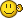## Recommended Posts

Hello autoit lovers. I am trying to find the total value of certain data within an ini file. Thanks in advance to anyone who can help. Bye.

CardID.ini

```[   0010171165  ]
Value1  =George Russell
Value2  =17
Value3  =120
Value4  =25

[   0010171588  ]
Value1  =Sergio Perez
Value2  =25
Value3  =100
Value4  =25

[   0010171166  ]
Value1  =Max Verstappen
Value2  =17
Value3  =85
Value4  =25

[   0010171524  ]
Value1  =Daniel Ricciardo
Value2  =17
Value3  =300
Value4  =0

[   0010171533  ]
Value1  =Lando Norris
Value2  =17
Value3  =102
Value4  =25

[   0010171534  ]
Value1  =Lance Stroll
Value2  =17
Value3  =340
Value4  =25

[   0010184458  ]
Value1  =Sebastian Vettel
Value2  =25
Value3  =80
Value4  =25```

GUİ Trial

```#include <GUIConstants.au3>

GUICreate("", 350, 700, -1, -1)
GUISetBkColor(0x00E0FFFF)

\$ltview = GUICtrlCreateListView("CARD-ID'S|NAME AND SURNAME|REMAIN", 10, 10, 330, 600) ;,\$LVS_SORTDESCENDING)
\$VButt = GUICtrlCreateButton("VALUE", 20, 630, 80, 20)
\$TButt = GUICtrlCreateButton("TOTAL", 120, 630, 80, 20)
\$input = GUICtrlCreateInput("", 220, 630, 80, 20)

GUICtrlSetState(-1, \$GUI_DROPACCEPTED)
GUISetState()

If Not @error Then
Dim \$item[UBound(\$nArray) + 1]
For \$i = 1 To UBound(\$nArray) - 1
If Not @error Then
\$V1 = ''
\$V3 = ''
For \$k = 1 To UBound(\$kArray, 1) - 1
If StringLower(\$kArray[\$k]) = 'Value1' Then \$V1 = \$kArray[\$k]
If StringLower(\$kArray[\$k]) = 'Value3' Then \$V3 = \$kArray[\$k]
\$Total = \$V3 + \$kArray[\$k]
Next
EndIf
\$item[\$i] = GUICtrlCreateListViewItem(\$nArray[\$i] &'|'& \$V1 &"|"& \$V3, \$ltview)
Next
EndIf

Do
\$msg = GUIGetMsg()
Select
Case \$msg = \$VButt
Case \$msg = \$TButt
GUICtrlSetData(\$input,\$Total)
EndSelect
Until \$msg = \$GUI_EVENT_CLOSE```##### Share on other sites

Total results of all data for example:

```#include <GUIConstants.au3>
Local \$iTotal = 0, \$vValue1, \$vValue3

GUICreate("", 350, 700, -1, -1)
GUISetBkColor(0x00E0FFFF)
Local \$idListView = GUICtrlCreateListView("CARD-ID'S|NAME AND SURNAME|REMAIN", 10, 10, 330, 600) ;,\$LVS_SORTDESCENDING)
Local \$idValue = GUICtrlCreateButton("VALUE", 20, 630, 80, 20)
Local \$idTotal = GUICtrlCreateButton("TOTAL", 120, 630, 80, 20)
Local \$idTotalInput = GUICtrlCreateInput("", 220, 630, 80, 20)

GUISetState()

If Not @error Then
For \$i = 1 To \$nArray
\$vValue1 = IniRead("CardID.ini", \$nArray[\$i], "Value1", "")
\$vValue3 = IniRead("CardID.ini", \$nArray[\$i], "Value3", "")
If Number(\$vValue3) > 0 Then \$iTotal += \$vValue3
GUICtrlCreateListViewItem(\$nArray[\$i] &'|'& \$vValue1 &"|"& \$vValue3, \$idListView)
Next
GUICtrlSetData(\$idTotalInput, \$iTotal)
EndIf

While 1
\$msg = GUIGetMsg()
Switch \$msg
Case \$GUI_EVENT_CLOSE
Exit
Case \$idValue
Case \$idTotal
GUICtrlSetData(\$idTotalInput,\$iTotal)
EndSwitch
WEnd```

## Create an account

Register a new account

• ### Recently Browsing   0 members

×

• Wiki

• Back

• #### Beta

• Git
• FAQ
×
• Create New...